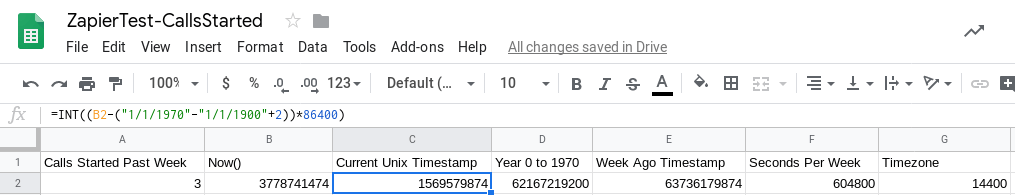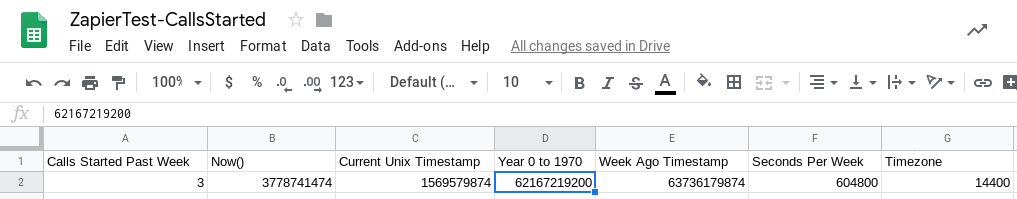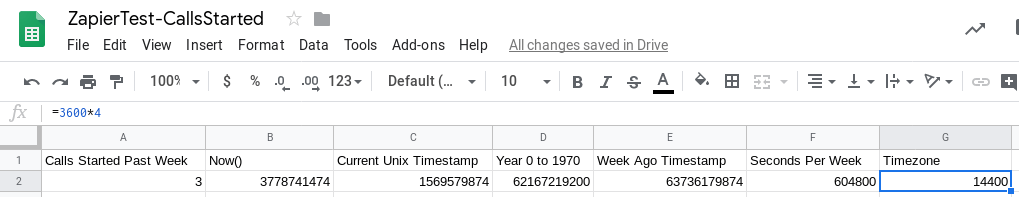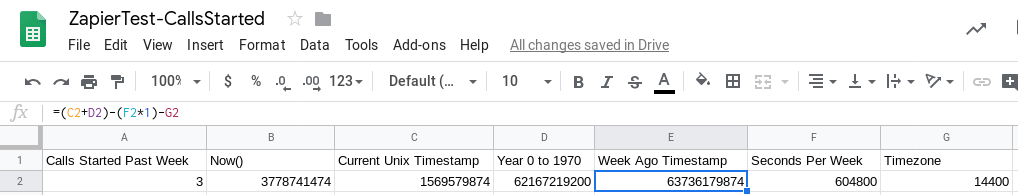# Use Google Sheets Formulas to Count Weekly Calls# Use Google Sheets Formulas to Count Weekly CallsDid you know that formulas in Google Sheets can make it easy to see department performance? Formulas are easy to learn and can help you summarize collections of data — like all the inbound calls you answer each week.

In today’s blog, we’ll give you a quick primer about Google Sheets formulas and show you how to calculate your weekly calls for use in company reports.

This post acts as a supplement to our other full Zapier tutorials, including Learn to Log VirtualPBX Calls in Google Sheets and Send Voicemail Data to Google Sheets.

Although we’ve provided you with a fully-working Sheet in the Preparation section of this blog, you can follow along with the directions below to understand how its formulas work.

## Preparation

### Copy Our Public Sheet

You can get started immediately by copying this Google Sheet. Open the link, then at the top of your screen, click File and then Make a copy to save the file to your own Google Drive.

Open the “Statistics” Worksheet of your Google Sheet. We’ll work through Columns B to G before addressing our count of weekly calls in Column A.

### Column B (The now() Function)

Finding the current timestamp in Google Sheets is easy. You can have a cell output that information by running the now() function, as shown in this example. Be aware that the timestamp shown here is the number days since Dec. 30, 1899. We will convert that figure into seconds and make other manipulations in future equations.### Column C (Current Unix Timestamp)

The Unix timestamp is a standard that counts the seconds since Jan. 1, 1970. It’s necessary to find the Unix time in order to understand the timestamps associated with VirtualPBX calls. Of all the Google Sheets formulas shown here, this may be the most confusing, but it’s definitely essential to the process.

Use this formula in Column C to create the Unix time from the Google Sheets time:

=INT((B2-(“1/1/1970”-“1/1/1900″+2))*86400)

The inner-most parenthesis here tell your Sheet to count the days between 1970 and 1899. Then it subtracts that number of days from the current time we found in Column B. After that, it multiplies the number of days by 86400, which is the number of seconds in a day.### Column D (Year 0 to 1970)

The way VirtualPBX outputs timestamps is by counting the seconds since Jan. 1, 0001 — the first day of A.D. In order to mix VirtualPBX logged values with the Unix timestamp (discussed in the previous section of this blog), we’ll need to add the number of seconds between that start date and Jan. 1, 1970.

This number of seconds — 62167219200 — is what’s shown in Column D of our Sheet.### Column F (Seconds Per Week) & G (Timezone)

Jumping ahead one column, to Column F, the value of 604800 is shown.

This is exactly what the column’s headline says: It’s the number of seconds in a week.Next, Column G includes a formula (=3600*4) to equal 14400. This value represents the shift of the number of seconds in each timezone. VirtualPBX logs calls in the GMT-4 timezone, so the number of seconds in one timezone (3600) is multiplied by 4 to reach the desired value.

Both these values will be used to calculate the Week Ago Timestamp shown in Column E.### Column E (Week Ago Timestamp)

Now the Week Ago Timestamp formula can make sense:

=(C2+D2)-(F2*1)-G2

It adds Columns C and D to produce the current timestamp (in seconds) when the log is accessed. This is analogous to the now() function shown in Column B but with a few transformations.

That value then subtracts the number of seconds in a week and compensates for the time zone change. You can change the value that Cell F2 is multiplied by to see the number of calls in 2, 3, 4… weeks. It would look something like (F2*2) to generate a timestamp from two weeks ago.### Column A (Calls Started Past Week)

Finally, we can see how many calls were started in the past week. The Google Sheets formula used here refers to the Calls Worksheet (tab) of the documents we provided in the Preparation section of this tutorial:

=countif(Calls!D2:D6, “>”&E2)

This formula tells your Sheet to look at Rows 2 through 6 in the Calls Worksheet. Those cells contain the timestamps of your logged calls. You can change the second value, D6, to analyze more calls. To that end, this formula would count the number of logged calls down to row 1000:

=countif(Calls!D2:D1000, “>”&E2)

No matter how many rows you count, this formula will compare each timestamp with the value of Cell E2 in our current Worksheet — Week Ago Timestamp. If the date of the call is newer than E2, it is counted. If the date is older, it isn’t counted.

The outputted value is an integer like you see in the screenshot here.